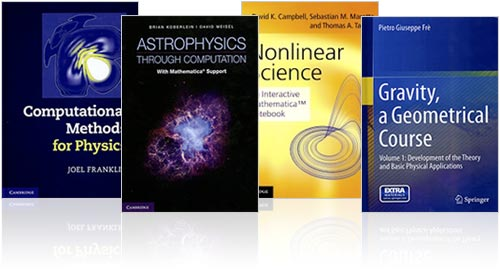Browse by Topic
Related Topics

# New Books Use Mathematica to Bring Math and Science to Life

The power, elegance, and interactivity of Mathematica are no secret to professionals and educators. Get your hands on some of these recent publications and find new ways to bring math and science to life in your workplace or classroom:Guide to Essential Math
This text is a highly focused, straightforward review of the necessary principles in math and physics needed for graduate school. Author Sy Blinder, who has over 40 years of teaching experience, provides clear examples, detailed illustrations, and relevant reference material compatible with Mathematica.

Nonlinear Science: An Interactive Mathematica Notebook
Using a Mathematica notebook as the medium, David K. Campbell, Sebastian M. Marotta, and Thomas A. Tanury have created an interactive teaching tool that lets its users explore firsthand the behavior of nonlinear systems. From coupled ODEs to nonlinear PDEs, this is a powerful resource for students, graduate students, and researchers in the field of nonlinear dynamics.

Astrophysics through Computation: With Mathematica Support
This textbook from Brian Koberlein and David D. Meisel uses Mathematica to allow readers to visualize principles of astrophysics in a new and engaging way. The book also analyzes the benefits of using computational problem-solving in astrophysics research.

Gravity, A Geometrical Course: Volumes 1 & 2
This two-book series takes a geometrical perspective on general relativity and gravitational theory. Author Pietro Giuseppe Frè transitions students away from special relativity, all the way into the beginnings of supergravity and superstring theory. Accompanying Mathematica packages and notebooks make it easy to explore topics addressed in the books.

Computational Methods for Physics
Computation is a foundational tool in physics. Joel Franklin presents this book as a guide to the most important techniques and methods for using Mathematica in typical physics problems. Supplemental exercises and reading material are also provided online.

Intelligent Routines: Solving Mathematical Analysis with Matlab, Mathcad, Mathematica and Maple
In this book by George A. Anastassiou and Iuliana F. Iatan, readers will have the opportunity to investigate the value of computational analysis on multiple software platforms, including Mathematica. Emphasis on problem-solving and conceptual understanding makes this textbook an ideal classroom resource.

Einführung in die Quantenphysik: Experimentelle und theoretische Grundlagen mit Aufgaben, Lösungen und Mathematica-Notebooks
(Introduction to Quantum Physics: Experimental and Theoretical Foundations with Exercises, Solutions, and Mathematica Notebooks)

This German-language book, by Peter Rennert, Angelika Chasse, and Wolfram Hergert, is an introduction to non-relativistic quantum mechanics. Approaching the topic from a mathematical perspective, this publication has detailed Mathematica problem sets and solutions provided for each chapter, with even more supplemental material available online.

Variable Compleja con Mathematica o Maxima
(Complex Variables with Mathematica or Maxima)

In this introductory mathematics book for complex variable analysis, José Ramírez Labrador provides enough material to cover two university semesters. The text, which is in Spanish, also includes supplementary practice problems for Mathematica.